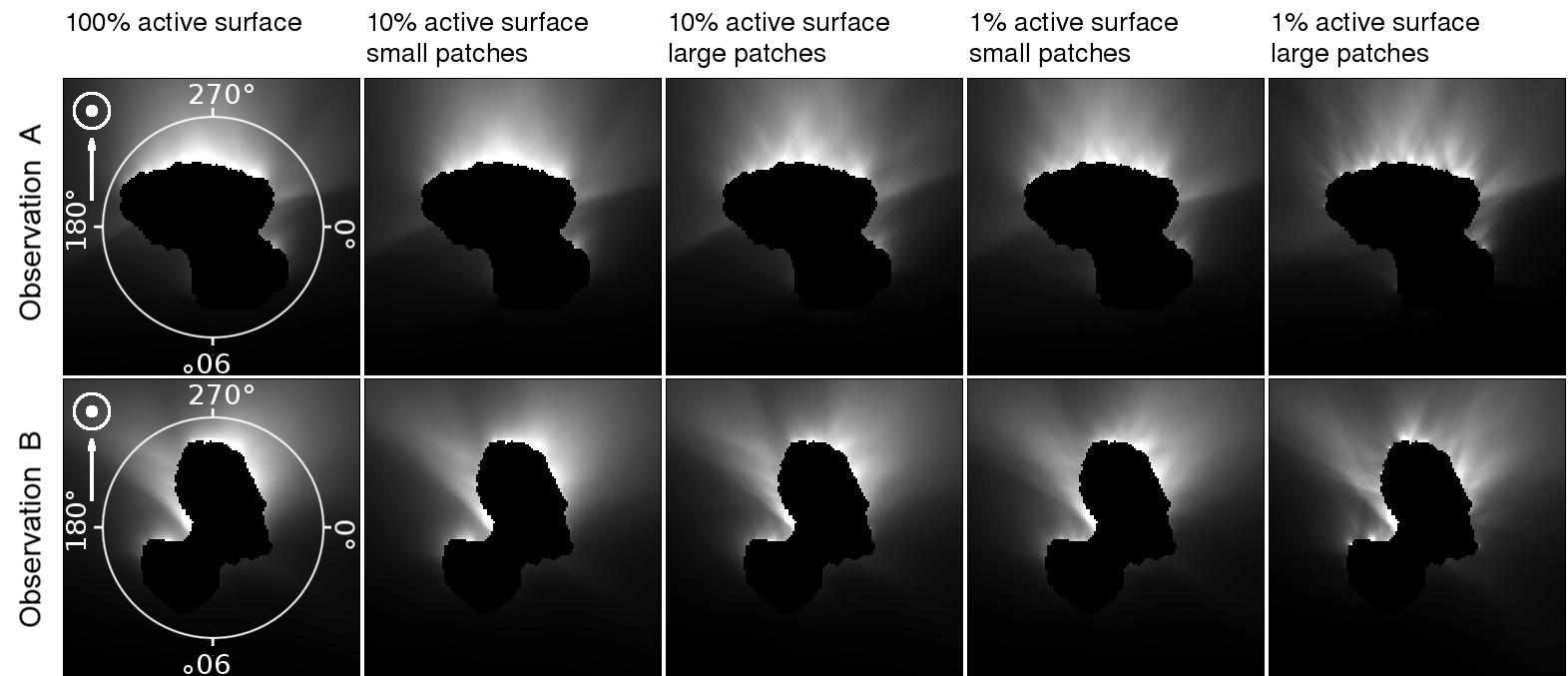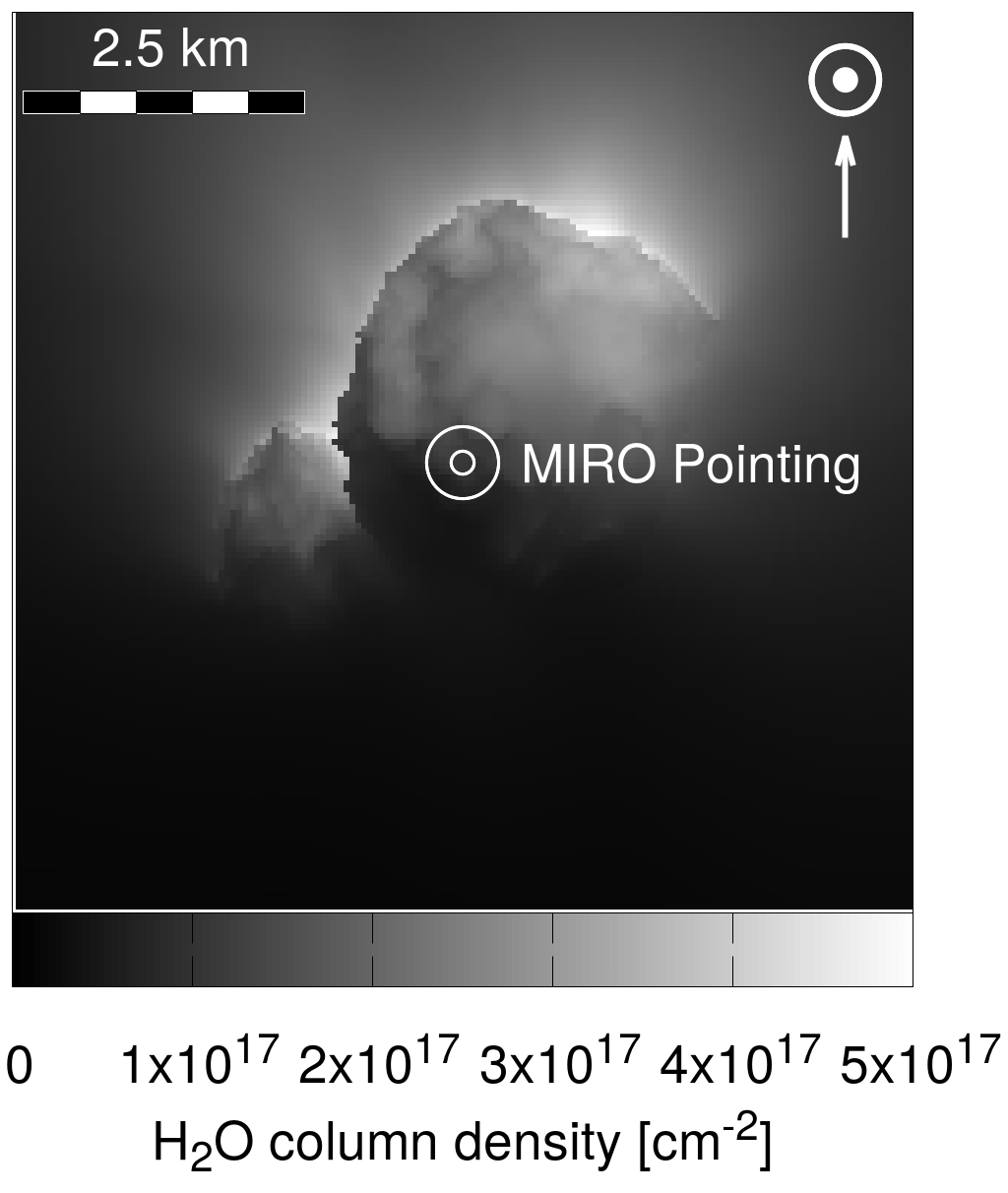Icarus, Volume 346, August 2020, 113742, https://doi.org/10.1016/j.icarus.2020.113742

## Limitations in the determination of surface emission distributions on comets through modelling of observational data – A case study based on Rosetta observations

#### Raphael Marschall, Ying Liao, Nicolas Thomas, Jong-Shinn Wu

This page contains interactive plots of the above-mentioned paper. Each interactive plot can be customised by changing the axis rages via the leavers at the top and right. By selecting different options in the legend the displayed data can be changed. Via the menu at the top left of each chart, the data can be downloaded and the current view saved as an image.

For all details on the figures please consult the paper. Here you can download the paperand supplementary figures. The abstract and a short summary of the modelling setup can be found below.

There are five interactive charts on this page comprising the following figures in the paper:

Figures 5, 6, B26-B35: Showing a ROSINA number density comparison of the 10 different models.

Figures 10 & 12: Showing a VIRTIS column density comparison for the 10 different models at two observation geometries.

Figures 13, 14, B36, B37: Showing a MIRO comparison of number density, gas speed, and temperature along the line of sight.

Figures 16 & B38: Showing a comparison between a homogeneous and a regionally inhomogeneous surface-emission distribution.

Figures 19 & B45: Showing a comparison of the fully collisional gas model to free molecular flow.

If you use the data or charts presented here please reference the paper: Marschall et al., Icarus, Volume 346, August 2020, 113742, https://doi.org/10.1016/j.icarus.2020.113742.

The number of data points has been reduced slightly compared to the paper for better web performance. Please contact me if you need the higher resolution data or have any questions.

#### Abstract

The European Space Agency’s (ESA) Rosetta mission has returned a vast data set of measurements of the inner gas coma of comet 67P/Churyumov-Gerasimenko. These measurements have been used by different groups to determine the distribution of the gas sources at the nucleus surface. The solutions that have been found differ from each other substantially and illustrate the degeneracy of this issue.

It is the aim of this work to explore the limitations that current gas models have in linking the coma measurements to the surface. In particular, we discuss the sensitivity of Rosetta’s ROSINA/COPS, VIRTIS, and MIRO instruments to differentiate between vastly different spatial distributions of the gas emission from the surface.

We have applied a state of the art 3D DSMC gas dynamics code to simulate the inner gas coma of different models that vary in the fraction of the surface that contains ice and in different sizes of active patches. These different distributions result in jet interactions that differ in their dynamical behaviour. We have then produced synthetic measurements of Rosetta’s gas instruments. By comparing the different models we probe the limitations of the different instruments to variations in the emission distribution.

We have found that ROSINA/COPS measurements by themselves cannot detect the differences in our models. While ROSINA/COPS measurements are important to constrain the regional inhomogeneities of the gas emission, they can by themselves not determine the surface-emission distribution of the gas sources to a spatial accuracy of better than a few hundred metres (≃400 m ~50 MFP). Any solutions fitting the ROSINA/COPS measurements is hence fundamentally degenerate, be it through a forward or inverse model. Only other instruments with complementary measurements can potentially lift this degeneracy as we show here for VIRTIS and MIRO. In particular, we find that MIRO is the only instrument that can distinguish between most of our models. Finally, as a by-product, we have explored the effect of our activity distributions on lateral flow at the surface that may be responsible for some of the observed aeolian features.

#### Simulation Setup

For comet 67P the global average active area fraction is only a few percents (typically 1%). This implies that the comet produces the amount of gas that is equivalent to 1% of the surface being a pure ice surface and the rest being inert. This naturally raises the question of how this low percentage is distributed on the surface and how we would detect this. We have thus devised a variety of extreme test cases to examine this exact question. Figure 2 illustrates the five basic distributions of ice we have tested. We have varied three basic parameters.Fig. 2: Five basic activity maps used in this map. Purple areas have the potential for outgassing with effective active fractions (EAF) that are non-zero, while green areas are fully inert, thus having an EAF of zero.

First, the percentage of the surface that has the potential for activity. The one extreme here is that the entire surface has 1% of ice coverage that is not resolved by our shape model (left column in Fig. 2). The other extreme is that 1% of the surface is pure ice with the remaining 99% of the surface being totally inert and hence having an active fraction of zero (right column in Fig. 2). We have also looked at the intermediate case where 10% of the surface is active (centre column in Fig. 2).

The second variable we have explored is how the ice is agglomerated on the surface. In the one extreme, we have used the smallest unit (the surface facets) acting as pure ice patches (bottom row in Fig. 2). In the other extreme, we have uniformly spaced larger patches (consisting of multiple facets) over the surface (top row in Fig. 2). For the 10% active surface case we have used 2000 large patches. In the 1% active surface case we have used 200 large patches were used.

The third variable we varied is the global production rate. We have performed two sets of simulations for each of the activity maps with a low global production rate of 2 kg/s (corresponding to approximately 3.0 AU inbound [~ November 16, 2014] and 2.8 AU outbound [~ April 9, 2016]) and a high global production rate 200 kg/s (corresponding to approximately 1.35 AU inbound [~ July 14, 2015] and 1.5 AU outbound [~ October 22, 2015]).

#### Observation geometries

We have produced synthetic measurements for three Rosetta gas instruments: ROSINA (Rosetta Orbiter Spectrometer for Ion and Neutral Analysis), VIRTIS (Visible and Infrared Thermal Imaging Spectrometer), and MIRO (Microwave Instrument for the Rosetta Orbiter).

ROSINA measured the local gas number density. We have extracted synthetic measurements along an artificial orbit. The sampling points are illustrated in Fig. 4. For phase angle steps every 5° synthetic measurements were taken with a 5° spacing along constant phase angle.Fig. 4: Illustration of the points along the artificial orbit from which gas number density measurements were taken to simulate what ROSINA/COPS would measure.

For VIRTIS we have compared the gas column density for two observation geometries with a phase angle of 60°. The observations geometries A and B can be seen in Figure 11 for the high activity case.Fig. 11: Synthetic VIRTIS-M-IR cubes for the five different models with a high global gas production rate for two different viewing geometries (A and B) at a phase angle of 60°.

For MIRO we have compared number densities, speeds along the line of sight (LOS) and temperature along the line of sight of the MIRO beam. A context image for the MIRO beam at a phase angle of 60° can be seen in Figure 13.Fig. 13: Shows a context image of the synthetic water column density and the pointing of the MIRO beam.

#### Figures 5, 6, B26-B35

Local gas number density [m-3] as a function of phase angle comparing the 100% (green), 10% (blue), and 1% (red) active surface models. All measurements have a 15% error associated with them but no error bars are drawn here for clarity. Measurements are taken every 5° in phase angle. The values between each 5° interval correspond to the measurements along the circle of constant phase angle (i.e. the values of the interval [5°, 10°) correspond to the measurements taken along the 5° circle). See Fig. 2 for activity distribution and Fig. 4 for orbit context in the simulation setup section above. The number of data points has been reduced slightly compared to the paper for a maximum of 25 data points per 5° phase angle.

#### Figures 10 & 12

Gas column density of the two observing geometries A and B for the 100% active area (green), 10% active area (blue), 1% active area (red) model as a function of the azimuth angle along the circles at 3 km from the nucleus centre indicated in Fig. 11. See Fig. 2 for activity distribution in the simulation setup section above. The number of data points has been reduced compared to the paper by a factor of two (one point every 2° instead of every 1°).

#### Figures 13, 14, B36, B37

The panels from left to right show the line of sight (LOS) profiles of the gas number density, temperature, and speed along the LOS as a function of altitude above the comet surface for a synthetic MIRO geometry at a phase angle of 60°. The three models of varying active area (100% in green, 10% in blue, and 1% in red). See Fig. 2 for activity distribution and Fig. 13 for the MIRO pointing in the simulation setup section above. The number of data points has been reduced compared to the paper to 30 point per decade in distance.

#### Figures 16 & B38

Local gas number density [m-3] as a function of phase angle comparing the cases of the 100% active surface models with homogeneous (blue) and Imhotep inhomogeneous (orange) ice distribu­tion. Measurements are taken every 5° in phase angle. The values between each 5° interval correspond to the measurements along the circle of constant phase angle (i.e. the values of the interval [5°, 10°) correspond to the measurements taken along the 5° circle). See Fig. 2 for activity distribution and Fig. 4 for orbit context in the simulation setup section above. The number of data points has been reduced slightly compared to the paper for a maximum of 25 data points per 5° phase angle.

#### Figures 19 & B45

Local gas number density [m 3 ] as a function of phase angle comparing the 100% active surface models assuming the full physics of colli­sions (blue) and free molecular flow (red) for the low gas production rate. Measurements are taken every 5° in phase angle. The values between each 5° interval correspond to the measurements along the circle of constant phase angle (i.e. the values of the interval [5°, 10°) correspond to the measurements taken along the 5° circle). See Fig. 2 for activity distribution and Fig. 4 for orbit context in the simulation setup section above. The number of data points has been reduced slightly compared to the paper for a maximum of 25 data points per 5° phase angle.